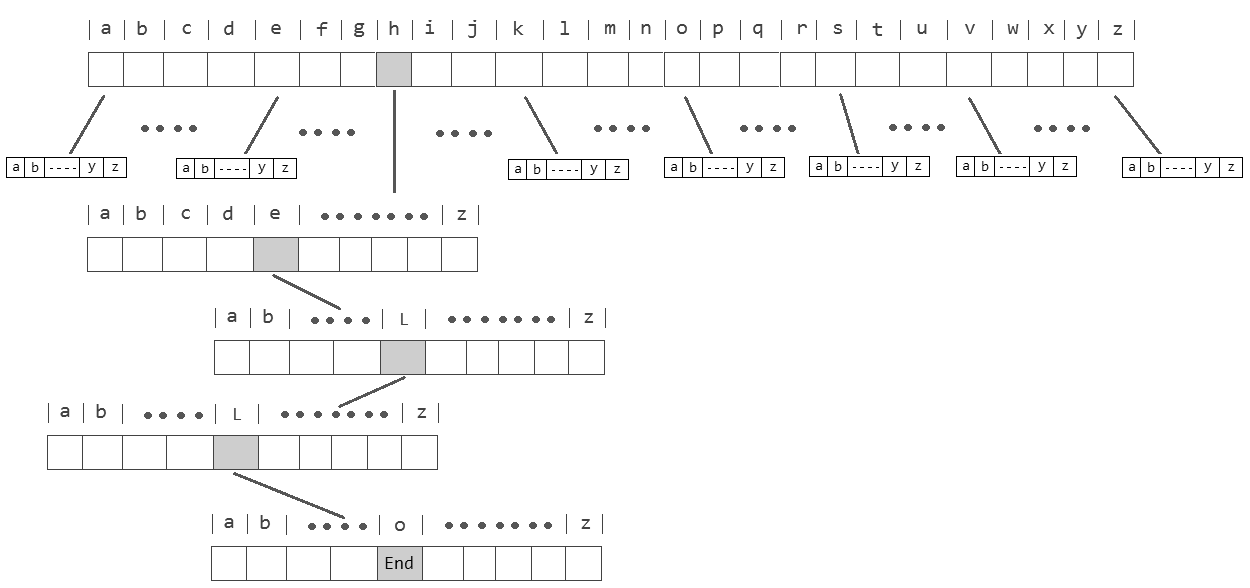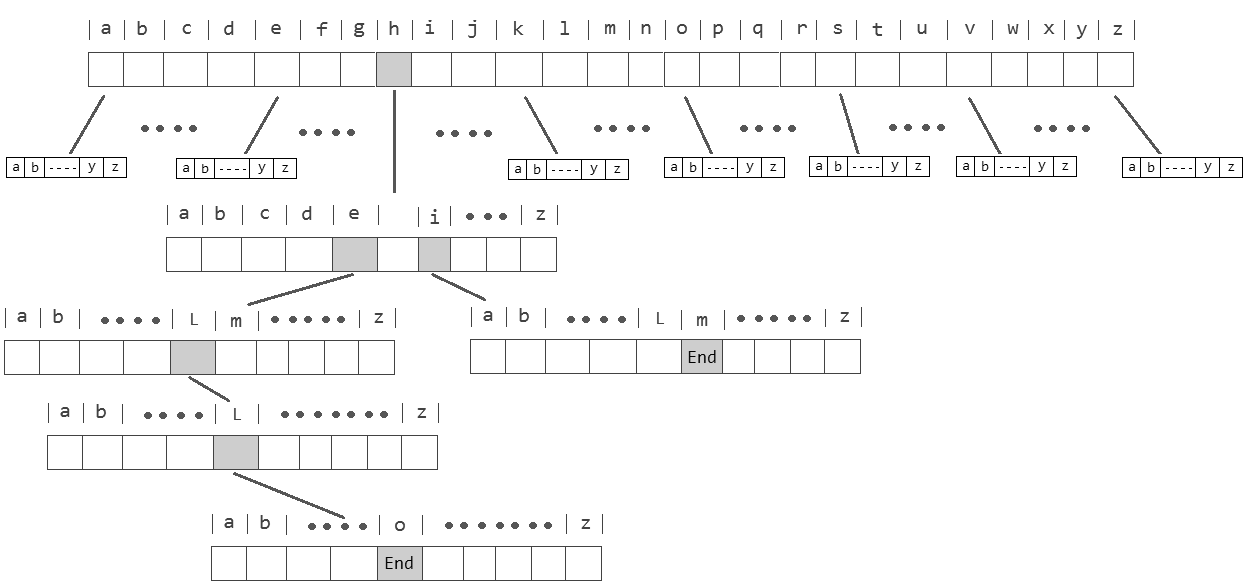# Implementing Immutable Trie in Scala

A Trie, (also called radix tree or prefix tree) is a kind of search tree to store a dynamic set or associative array where the keys are usually String. Each Trie starts with an empty node as the root. Every node consists of multiple branches. Each branch represents a possible character of keys.

For defining the branches they usually have an array with a fixed size for all possible characters. For example, if we are representing the English alphabet, then the total number of child nodes will be 26. (English alphabet contains 26 letters). So for storing a word, we need to take an array(container) of size 26 and initially, all the characters are blank as there are no words and it will look as shown below:

Let’s store the words “hello” and “him”. “hello” starts from “h”, So we will mark the position “h” as filled, which represents the use of “h”.
After placing the first character, for the 2nd character again there are 26 possibilities, So from “h”, again there is an array of size 26, for storing the 2nd character.

The second character is “e”, So from “h”, we will move to “e” and mark “e” in the 2nd array as used.
After “e”, the 3rd character is “l”, So mark the position “l” as used in the respective array.

Repeat the above steps for all the characters of a word and Trie will look like below.A Trie which contains only hello

Now let’s add “him” to the tree, “him” starts from “h”, So we will mark the position “h” as filled, but “h” is already filled by the word “hello” which indicates that there exists some other word starting with “h”.
Using the same position “h” as a reference, Now, for the 2nd character “i”, We will move to “i” and mark position “i” in the 2nd array as used. This signifies, that there exist 2 words starting with “he” and “hi”.
Now comes 3rd character “m” after “i”, Now, we need to start new 26 array alphabet set again, which will hold the series starting from “hi”. So mark the position “m” as used in a respective array.

After storing “hello” and “him”, Trie will look like below.A Trie which contains hello and him keys

Read more about binary trees on Wikipedia.

Implementation: I will define a case class for Node that will store one optional value and a List of children.

`case class Trie[V](value: Option[V],children: List[Option[Trie[V]]])`

For creating the empty tree we can define an apply method in the companion object, for each Node we need to fill the children array with empty values.

`object Trie {  def empty[V]: Trie[V] = new Trie[V](None, List.fill(26)(None))  def apply[V]: Trie[V] = empty[V]}`

At least three operations should be done with this tree:

• `insert` which inserts a new key/value and yield a new updated Trie
• `delete` which removes a key/value if it exists and creates a new Trie
• `search` which returns an `Options[V]`

Here you can see the whole implementation which supports `insert` `delete` and `search` operations.

Here it is in action:

As you can see the downside of a Trie is memory consumption, because it takes up a lot of memory and space with empty values (`None` ).

In Scala `Vector` internally uses a Trie structure, (A 32-way Trie), which each node uses Array and store either 0–32 references to child nodes. this number is called `Branching Factor`.

### Ternary Search Tree

A `Ternary Search Tree` is a special Trie data structure which resolves the memory consumption of the Trie data structure, in this tree each node has only three children. Left and Right which are the same as in Binary search tree. Mid child contains the next letter of the word which parent is part of.

A TST node has five fields:

• A field to store the value of the key.
• A field to store the char
• The left pointer points to the node whose char value is less than the value in the current node.
• The equal pointer points to the node whose char is equal to the value in the current node.
• The right pointer points to the node whose char is greater than the value in the current node.

For the implementation, I define a `sealed trait` a case class for Node and a case object for the Leaf nodes.

`sealed trait Ternary[+A]`
`case class Node[V](value: Option[V], char: Char, left: Ternary[V], mid: Ternary[V], right: Ternary[V]) extends Ternary[V]                                               `
`case object Leaf extends Ternary[Nothing]`

Let’s see an immutable implementation of Ternary Search Tree data structure.

Here it is in action.

This data structure is efficient for queries like “Given a word, find the next word in the dictionary” (Autocomplete feature). The implementation contains a function `keys` which return all keys in sorted order and `keysWithPrefix` which finds all the keys in the tree starting with a given prefix.

References

1. Algorithms: Tries, Robert Sedgewick and Kevin Wayne
2. Trying to Understand Tries